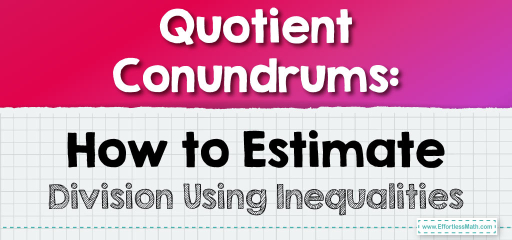# Quotient Conundrums: How to Estimate Division Using Inequalities

When dividing numbers, especially in complex scenarios, it's often helpful to know the range within which the quotient lies. Using inequalities, we can provide an estimated range for the quotient, offering a clearer picture of the possible outcomes. Let's delve into how to estimate quotients using inequalities.## Estimating Quotients with Inequalities

### Example 1:

Estimate the quotient range for $$315$$ divided by $$28$$.

Estimation Process:

1. Round $$315$$ down to $$300$$ and up to $$320$$.

2. Round $$28$$ down to $$20$$ and up to $$30$$.

3. Calculate the lower estimate: $$300 \div 30 = 10$$.

4. Calculate the upper estimate: $$320 \div 20 = 16$$.

The estimated quotient is between $$10$$ and $$16$$, or $$10 \leq q \leq 16$$.

The Absolute Best Book for 5th Grade Students

### Example 2:

Estimate the quotient range for $$642$$ divided by $$53$$.

Estimation Process:

1. Round $$642$$ down to $$640$$ and up to $$650$$.

2. Round $$53$$ down to $$50$$ and up to $$60$$.

3. Calculate the lower estimate: $$640 \div 60 = 10.67$$. Round this to $$10$$.

4. Calculate the upper estimate: $$650 \div 50 = 13$$.

The estimated quotient is between $$10$$ and $$13$$, or $$10 \leq q \leq 13$$.

Estimating quotients using inequalities provides a range of possible values, making it easier to gauge the outcome without exact calculations. This method is especially useful when you need a ballpark range for planning, budgeting, or making informed decisions. With practice, you’ll become adept at quickly estimating quotient ranges using inequalities!

### Practice Questions:

1. Estimate the quotient range for $$528$$ divided by $$47$$.

2. What is the estimated quotient range when $$870$$ is divided by $$58$$?

3. Divide $$903$$ by $$64$$ and estimate the quotient range.

4. What is the estimated range for $$350$$ divided by $$29$$?

5. Estimate the quotient range for $$1210$$ divided by $$44$$.

A Perfect Book for Grade 5 Math Word Problems!

1. $$11 \leq q \leq 16$$

2. $$15 \leq q \leq 17$$

3. $$14 \leq q \leq 18$$

4. $$12 \leq q \leq 17$$

5. $$27 \leq q \leq 30$$

The Best Math Books for Elementary Students

### What people say about "Quotient Conundrums: How to Estimate Division Using Inequalities - Effortless Math: We Help Students Learn to LOVE Mathematics"?

No one replied yet.

X
51% OFF

Limited time only!

Save Over 51%

SAVE $15 It was$29.99 now it is \$14.99